# Gravity Questions and Answers (Q&A)#### BHumberdross

The statement that best-described gravity has to be 1. This is the force that pulls the objects to the ground. It should be remembered that gravity is known to be a force that pulls objects towards the center of the earth. This applies to anything that has mass. Take note that if there are other laws that are considered, then there may be slight changes to the rule.

Over the past years, there are some changes to this description namely that gravity can be because of the attraction between two different objects that have masses. It is probable that there are still going to be other changes about that in the long run.#### I. Klose

Because it is. Let me explain!

Newton’s concept of gravity is good for explaining the basic motions around us and still holds in many situations but the problem is that Newton made a mistake. Newton treated gravity as a force, and in working, gravity isn’t a force which accelerates. Scientifically speaking, gravity doesn’t exist like Newton defines it. Newton was never able to explain how gravity works and refused to even try.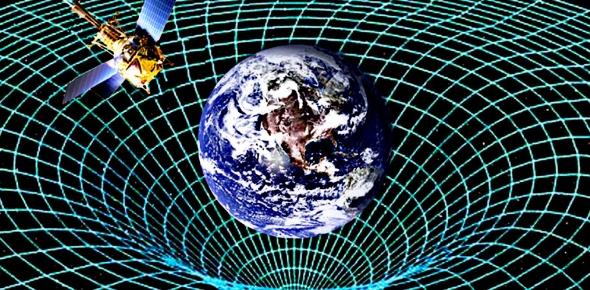Einstein concluded that gravity is something you ‘feel’ when you have a surface. Once that surface is removed, there is no gravity and you are weightless. All objects in the universe are simply free falling towards the deep hollow of the universe between space and time, and external barriers and forces like velocity, magnetic fields etc keep them from doing so. Gravity is simply a curvature of spacetime. For example, we are falling into the center of the earth because of its mass and we would keep doing it but the surface of the earth prevents us from doing so. The surface acts as a barrier and causes a curvature in spacetime. This curvature is the gravity we feel.

Newtonian gravity holds in 3 dimensions but fails when the 4th dimension of time is added. Newton’s theory treats time as a constant whereas Einstein defines gravity in a 4d space defining time as another dimension. Einstein’s definition holds true both experimentally and theoretically and is therefore considered more accurate.

Newton would be so pissed if he was alive!#### I. Klose

Before discussing gravitational radiation, let’s talk gravitational waves, as they play a huge role in gravitational radiation. Gravitational waves are created when there is a disturbance in space and time, which creates curvature that radiates outward from the center of accelerated masses. Mass is what causes these waves to curve outward from the source, and the more mass within a certain volume of space then there is more curvature seen in the waves seen at the boundary of space and time.
These curves change as the objects (white dwarfs, neutron stars, or black holes collisions) move around reflecting that the objects have moved. Sometimes, when objects accelerate, the curve will also move outward at the speed of light in a wave-like manner. These activities are referred to as gravitational waves.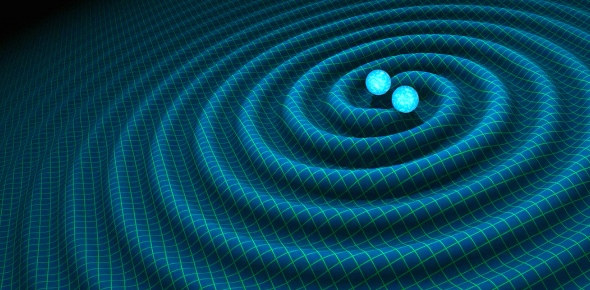Everything creates energy, regardless of what it is, and in this case, these waves create energy. When massive bodies move quickly, radiant energy is created, but because gravity is weak, it can only be detected when intense astrophysical events occur, i.e. black holes colliding and supernovae. The energy that is produced by these waves that occur when massive bodies collide is referred to as gravitational radiation.#### I. Klose

Gravity is considered to be an inherent part of everybody because everything that can be seen in this world is connected to the center which is where gravity is supposed to come from. People are being pulled towards the center of the earth. There are also times when some people and items will be pulled more by gravity usually when things are very heavy.

When you throw something to the air, you can expect that it will fall down eventually. The rate that it is going to fall down will depend on the weight of the items. There was a time when people have assumed that gravity can be found within but because of further studies, it became connected to the earth’s center.#### I. Muller

Yes, this is a curious feature about the Hudson Bay area of Canada. There is a degree less gravity, as was discovered in the 1960s when scientists were charting the Earth's global gravity fields. They discovered found a weakness, although slight.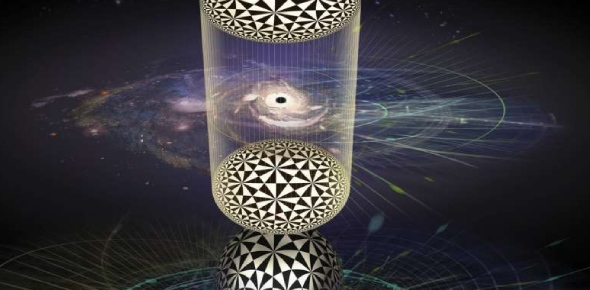This would amount to something like a tenth of an ounce for someone weighing 150-pound. There are two explanations for this phenomenon: convection in the Earth's mantle, and the melting of the Laurentide Ice Sheet.#### I. Muller

This is a theory still being developed in physics that attempts to take account of both quantum mechanics and general relativity. If a successful description of quantum gravity could be achieved, then this might amount to a 'theory of everything' - no small matter!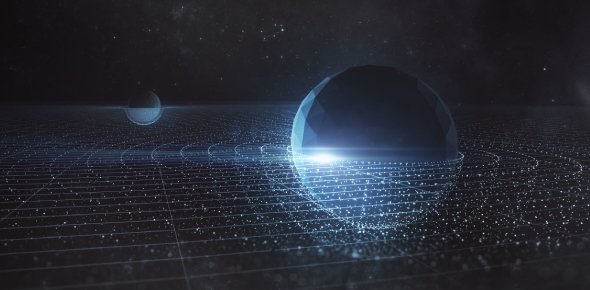Quantum gravity is an overall term for such combinational theories, ie attempts to unify gravity with the other fundamental forces of physics. It usually amounts to proposing the existence of a theoretical entity, a graviton, which is a virtual particle that mediates the gravitational force.#### I. Muller

The discussion of gravitational singularities must be considered as a theorem. In two hypothetical situations, the implosion of a planetary body or the universe spatially closed. In either case, the laws of physics as we know them cease to operate and gravitational singularities would be expected.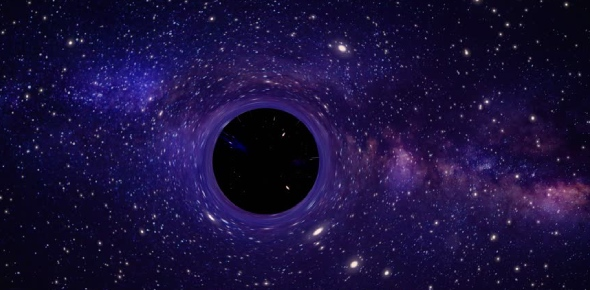In the center of a black hole is a gravitational singularity. this is a one-dimensional point which contains a huge mass but in an infinitely small space. Here density and gravity become infinite so that space-time curves infinitely. Our known laws of physics cannot encompass such eventuality.#### I. Muller

Super-gravity is a type of quantum theory. This theory includes consideration of gravity along with the other fundamental forces. These other three are the electromagnetic force, the weak nuclear force, and the strong nuclear force.. Super gravity is a field theory proposing that elementary subatomic particles and their interactions are based on the particle symmetry.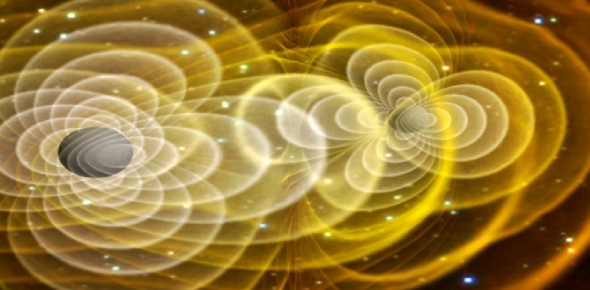This is also known as supersymmetry and that includes the gravitational force along with the other fundamental interactions of matter. In essence, super-gravity combines the principles of supersymmetry and of general relativity into one field theory.#### I. Klose

The sun's gravity is 28 times that of the Earth's. The more mass an object has, the stronger its gravity will be. Therefore the sun attracts the Earth towards it and the sun's gravity is responsible for the Earth's regular orbit through the solar system.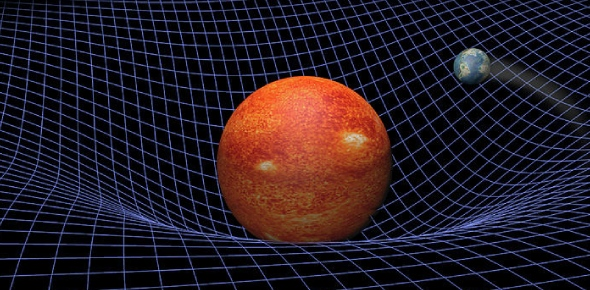The Earth travels at a velocity that approximates the force the sun exerts on the planet, resulting in a regular orbit. The variation in the strength of the sun's gravitational pull from the side of the earth facing towards the sun to the part facing away is partly responsible for the tides of the ocean.#### I. Muller

Gravitational waves differ from gravity waves. Gravitational waves are ripples in space-time that travel outward from a source. In the twentieth century the existence of gravitation waves was proved, but not directly observed. The theory explains that a massive object like Earth distorts space-time around it The larger the object, the more space-time is distorted as a direct result of that object's presence.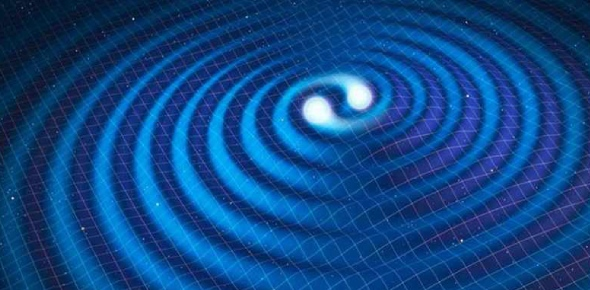One illustrative explanation is that if a marble were circling around the bowling ball on the dimpled trampoline, the marble would fall inward, toward the bowling ball, like a rock in space circling a planet.